## Parabolic Coordinates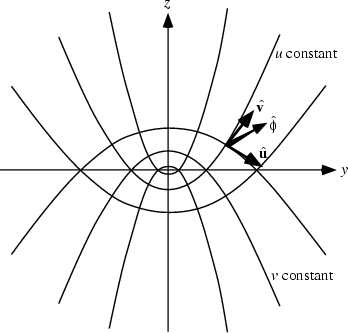A system of Curvilinear Coordinates in which two sets of coordinate surfaces are obtained by revolving the parabolas of Parabolic Cylindrical Coordinates about the x-Axis, which is then relabeled the z-Axis. There are several notational conventions. Whereas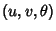is used in this work, Arfken (1970) uses.

The equations for the parabolic coordinates are(1)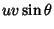(2)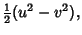(3)

where,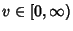, and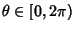. To solve for,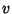, and, examine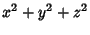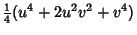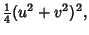(4)

so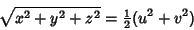(5)

and(6)(7)

We therefore have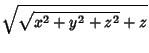(8)(9)(10)

The Scale Factors are(11)(12)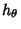(13)

The Line Element is(14)

and the Volume Element is(15)

The Laplacian is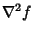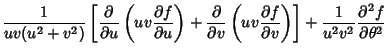(16)

The Helmholtz Differential Equation is Separable in parabolic coordinates.

See also Confocal Paraboloidal Coordinates, Helmholtz Differential Equation--Parabolic Coordinates, Parabolic Cylindrical Coordinates

References

Arfken, G. Parabolic Coordinates (,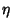,).'' §2.12 in Mathematical Methods for Physicists, 2nd ed. Orlando, FL: Academic Press, pp. 109-112, 1970.

Morse, P. M. and Feshbach, H. Methods of Theoretical Physics, Part I. New York: McGraw-Hill, p. 660, 1953.